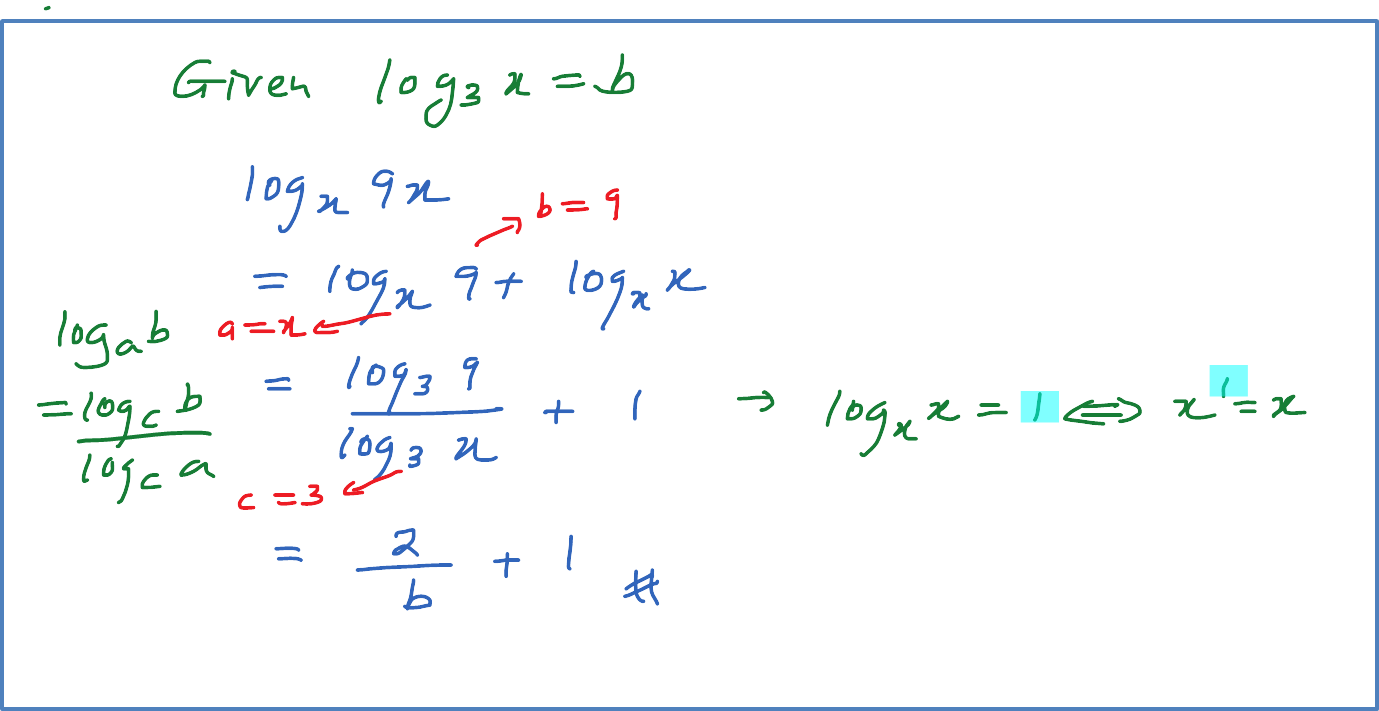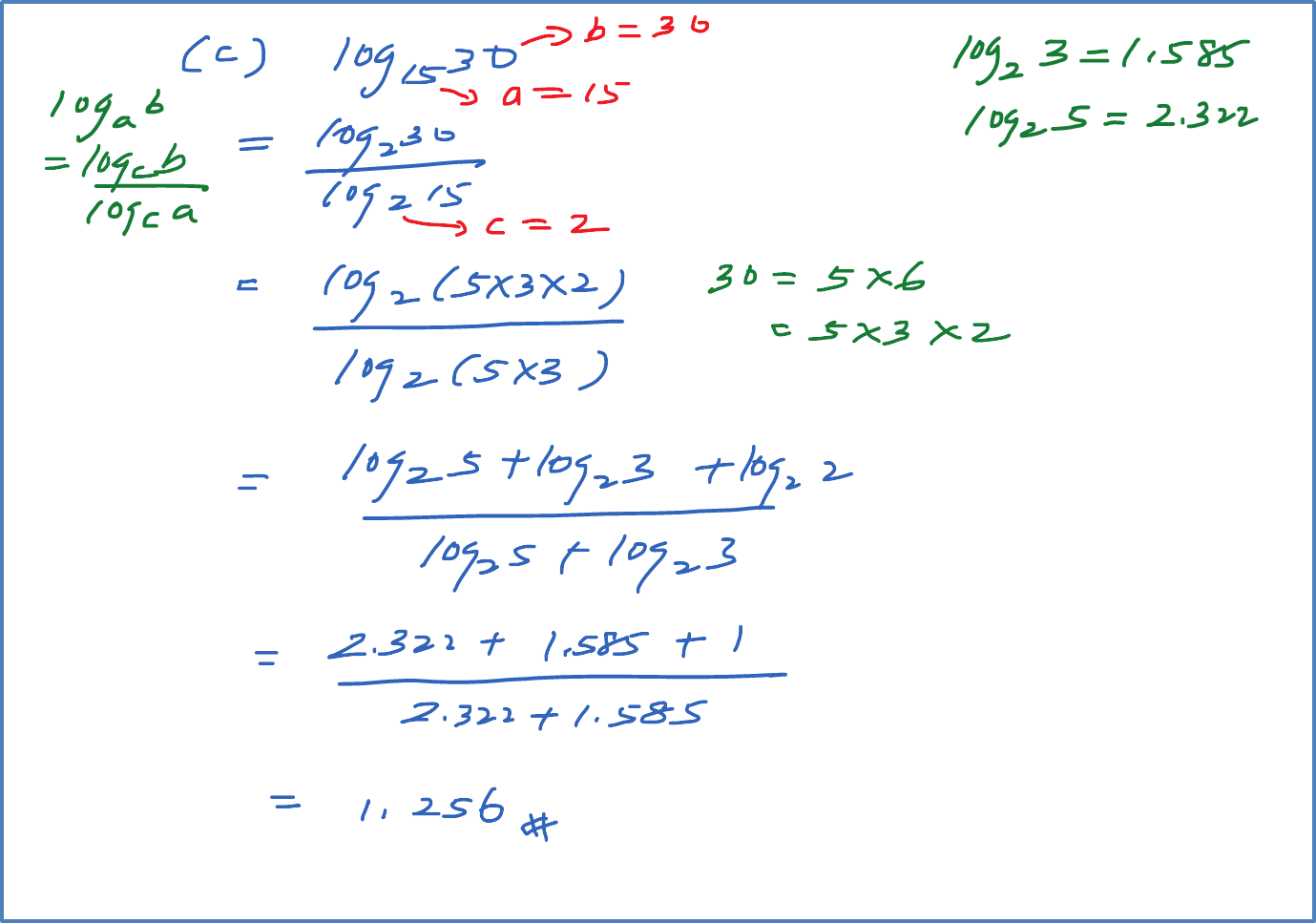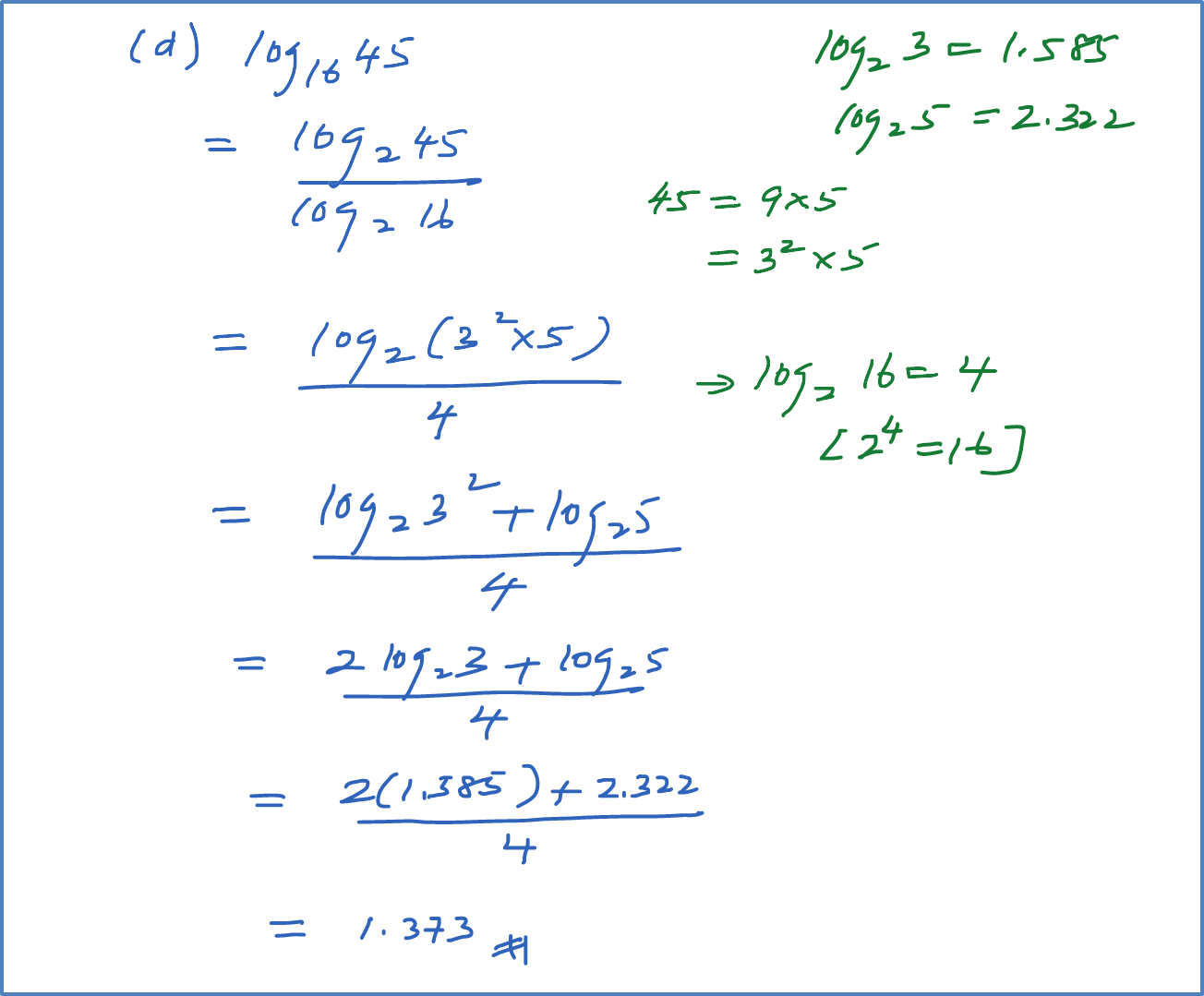\

# 4.2.2(c) Example 3 (Change of Base of Logarithms)

Example 3
Given that ${\mathrm{log}}_{3}x=b$  , express ${\mathrm{log}}_{x}9x$ in term of b.Example 4
Given that ${\mathrm{log}}_{2}3=1.585$   and ${\mathrm{log}}_{2}5=2.322$  , evaluate the following.
(a) ${\mathrm{log}}_{8}15$
(b) ${\mathrm{log}}_{5}0.6$
(c) ${\mathrm{log}}_{15}30$
(d) ${\mathrm{log}}_{16}45$

Solution: